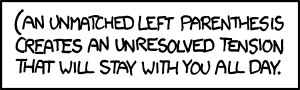시간 제한메모리 제한제출정답맞은 사람정답 비율
2 초 512 MB111100.000%

## 문제balanced bracket sequence is a string consisting only of the characters "(" (opening brackets) and ")" (closing brackets) such that each opening bracket has a "matching" closing bracket, and vice versa. For example, "(())()" is a balanced bracket sequence, whereas "(())(()" and "())(()" are not.

Given two bracket sequences A and B of the same length, we say that A is lexicographically smaller than B (and write A < B) if:

1. A and B differ in at least one position, and
2. A has a "(", and B has a ")" in the left-most position in which A and B differ

For example "(())()" < "()()()" because they first differ in the second position from the left, and the first string has an "(" in that position, whereas the second string has a ")". For a given length N, the "<" operator defines an ordering on all balanced bracket sequences of length N. For example, the ordering of the sequences of length 6 is:

1. ((()))
2. (()())
3. (())()
4. ()(())
5. ()()()

Given a length N and a positive integer M, your task is to find the Mth balanced bracket sequence in the ordering.

## 입력

You will be given an even integer N (2 ≤ N ≤ 2000), and a positive integer M. It is guaranteed that M will be no more than 1018 and no more than the number of balanced bracket sequences of length N (whichever is smaller).

## 출력

Output the Mth balanced bracket sequence of length N, when ordered lexicographically.

## 예제 입력 1

6 4


## 예제 출력 1

()(())